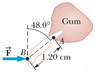# Rotational Motion and Law of Gravity

a) Two objects attract each other with a gravitational force of magnitude 9.30 x10-9 N when separated by 19.4 cm. If the total mass of the objects is 5.06 kg, what is the mass of each?

Heavier mass_______kg
Lighter mass______ kg

my attempt:
F=Gm1m2/r^2
so I rearranged to get m1 and m2 by itself and got (r^2)(F)/G=m1m2
5.247598=m1m2
but how do I get the mass for m1 and m2 separately is m1 + m2 = 5.06 kg?

b) A dental bracket exerts a horizontal force of 86.2 N on a tooth at point B in the figure. What is the torque on the root of the tooth about point A? (PLEASE SEE THE ATTACHMENT FOR THE PICTURE)

I know I need to break the forces up into components but I am lost on this question. Please help me! Thank you

#### Attachments

•torque.gif
4 KB · Views: 515
a) Two objects attract each other with a gravitational force of magnitude 9.30 x10-9 N when separated by 19.4 cm. If the total mass of the objects is 5.06 kg, what is the mass of each?

Heavier mass_______kg
Lighter mass______ kg

my attempt:
F=Gm1m2/r^2
so I rearranged to get m1 and m2 by itself and got (r^2)(F)/G=m1m2
5.247598=m1m2
but how do I get the mass for m1 and m2 separately is m1 + m2 = 5.06 kg?

Yes, the total mass is given as 5.06 kg, so m1 + m2 = 5.06 kg. Replace one of the masses, say m2, with m2 = (5.06 kg - m1).

I used this and found my answer to be .433 and 4.627 but its wrong. Do i have my formula correct?

I used this and found my answer to be .433 and 4.627 but its wrong. Do i have my formula correct?

Your formula (Newton's law of gravitation) looks okay. Perhaps something went wrong in your math manipulations. If you post your calculations they can be checked.

actually I just solved it! It was just a silly error! But thank you for your help

Do you have nay idea how to do part b ?